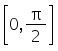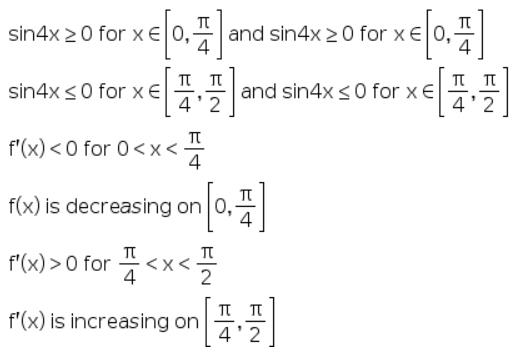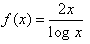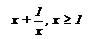# Test: Increasing And Decreasing Functions

## 5 Questions MCQ Test Mathematics (Maths) Class 12 | Test: Increasing And Decreasing Functions

Description
This mock test of Test: Increasing And Decreasing Functions for JEE helps you for every JEE entrance exam. This contains 5 Multiple Choice Questions for JEE Test: Increasing And Decreasing Functions (mcq) to study with solutions a complete question bank. The solved questions answers in this Test: Increasing And Decreasing Functions quiz give you a good mix of easy questions and tough questions. JEE students definitely take this Test: Increasing And Decreasing Functions exercise for a better result in the exam. You can find other Test: Increasing And Decreasing Functions extra questions, long questions & short questions for JEE on EduRev as well by searching above.
QUESTION: 1

### Separate the intervalinto sub-intervals in which f (x) = sin4 x + cos4 x is increasing or decreasing

Solution:

f’(x) = 4sin3x cosx - 4cos3x sinx
= - sin4x
As x is in the interval [0, ∏/2] hence 4x is in the interval [0, 2∏]QUESTION: 2

### The function f(x) = ax, 0 < a < 1 is​

Solution:

f(x) = ax
Taking log bth the sides, log f(x) = xloga
f’(x)/ax = loga
f’(x) = ax loga   {ax > 0 for all x implies R,
for loga e<a<1 that implies loga < 0}
Therefore, f’(x) < 0, for all x implies R
f(x) is a decreasing function.

QUESTION: 3

### The functionincreases in​

Solution:

f'(x) = [(logx).2−2x.1/x](logx)2
= 2(logx−1)/(logx)^2
∴f'(x)>0
⇔logx−1>0
⇔logx>1
⇔logx>loge
⇔x>e
∴f(x) is increasing in (e,∞)

QUESTION: 4

The function f (x) = -3x + 12 on R.is​

Solution:

f(x) = -3x + 12
f(0) = -3(0) + 12 = 0 - 12 = 0
f(1) = 9
f(2)= 6
f(3) = 3
f(4) = 0
f(5) = -3

QUESTION: 5

The functionis​

Solution:

f(x) = x + 1/x
f’(x) = 1 - 1/x2
= (x2 - 1)/x2
= [(x - 1) (x + 1)]/x2
= x ≥ 1
Therfore, x - 1 ≥ 0,   x + 1 ≥ 0
[(x - 1) (x + 1)]/x2 ≥ 0
f’(x) ≥ 0  => f(x) is increasing.